# How to Calculate the Sides and Angles of Triangles

Eugene is a qualified control/instrumentation engineer Bsc (Eng) and has worked as a developer of electronics & software for SCADA systems.

## Trigonometry and Solving Triangles

In this tutorial, you'll learn about trigonometry which is a branch of mathematics that covers the relationship between the sides and angles of triangles.

We'll find out about:

• Polygons and the Definition of a Triangle
• The Basic Facts About Triangles
• The Triangle Inequality Theorem
• Different Types of Triangles
• Using the Greek Alphabet for Equations
• Sine, Cosine and Tangent
• Pythagoras's Theorem
• The Sine and Cosine Rules
• How to Work Out the Sides and Angles of a Triangle
• Measuring Angles
• How to Calculate the Area of a Triangle

## What Is a Triangle?

By definition, a triangle is a polygon with three sides.

Polygons are plane shapes with several straight sides. "Plane" just means they're flat and two-dimensional. Other examples of polygons include squares, pentagons, hexagons and octagons. The word plane originates from the Greek polús meaning "many" and gōnía meaning "corner" or "angle." So polygon means "many corners." A triangle is the simplest possible polygon, having only three sides.Polygons with different numbers of sides. Regular polgons have sides the same length.© Eugene Brennan

## Basic Facts About Triangles

• A triangle is a polygon with three sides.
• All the internal angles add up to a total of 180 degrees.
• The angle between two sides can be anything from greater than 0 to less than 180 degrees.
• The angle between two sides can't be 0 or 180 degrees, because the triangle would then become three straight lines superimposed on each other (These are called degenerate triangles).
• Similar triangles have the same angles, but different length sides.

## The Symbol for Degrees

Degrees can be written using the symbol º. So, 45º means 45 degrees.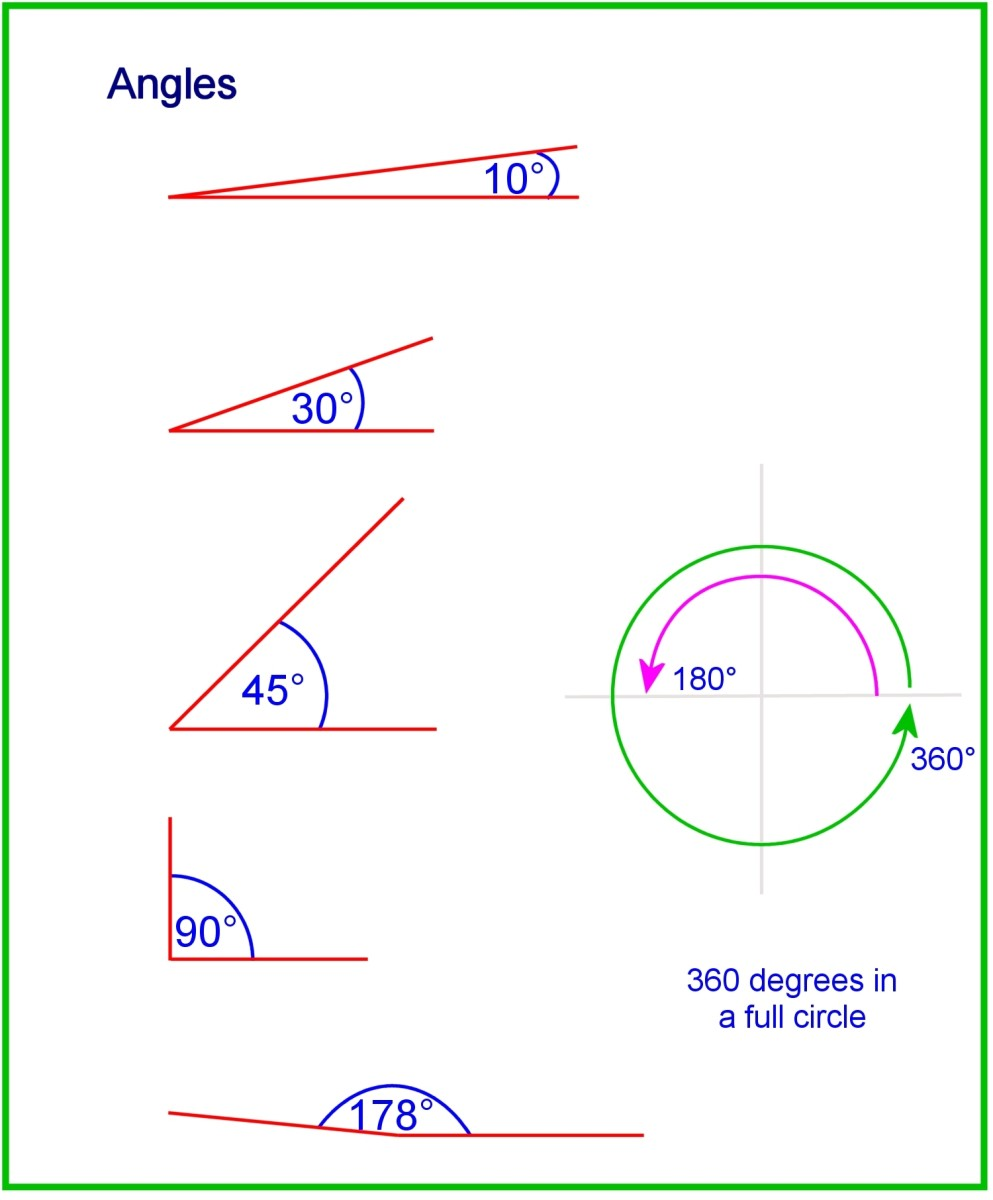Angles of a triangle range from 0 to less than 180 degrees.© Eugene Brennan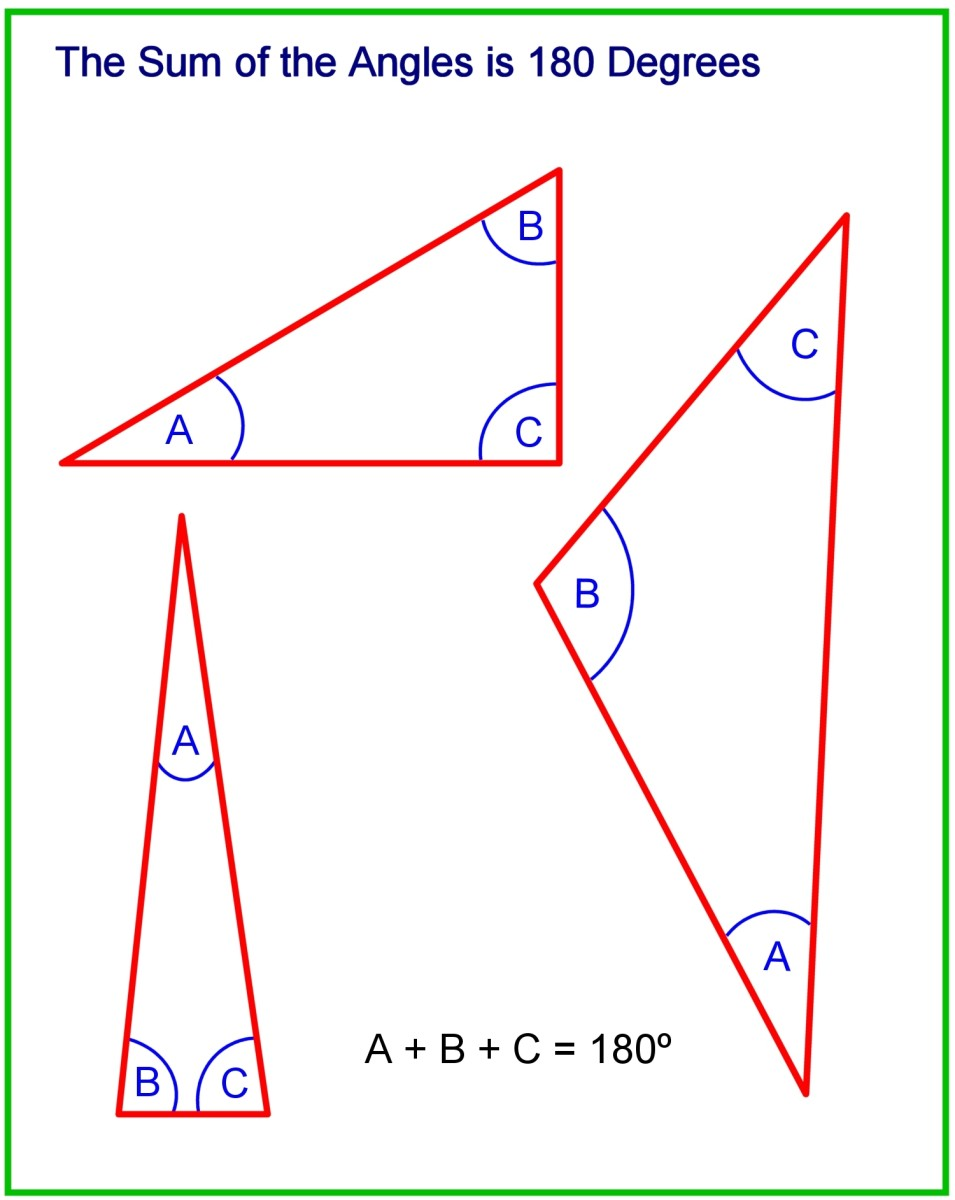No matter what the shape or size of a triangle, the sum of the 3 internal angles is 180 © Eugene Brennan

## What Is the Triangle Inequality Theorem?

This states that the sum of any two sides of a triangle must be greater than or equal to the remaining side.

## What Are the Different Types of Triangles?

Before we learn how to work out the sides and angles of a triangle, it's important to know the names of the different types of triangles. The classification of a triangle depends on two factors:

Scroll to Continue

## Read More From Owlcation

• The length of a triangle's sides
• The angles of a triangle's corners

Types of triangles by length of sides.

Type of Triangle by Lengths of SidesDescription

Isosceles

An isosceles triangle has two sides of equal length, and one side that is either longer or shorter than the equal sides.

Equilateral

All sides and angles are equal in length and degree.

Scalene

All sides and angles are of different lengths and degrees.

Types of triangles by angle.

Type of Triangle by Internal AngleDescription

Right (right angled)

One angle is 90 degrees.

Acute

Each of the three angles measure less than 90 degrees.

Obtuse

One angle is greater than 90 degrees.

## Using the Greek Alphabet for Equations

Another topic that we'll briefly cover before we delve into the mathematics of solving triangles is the Greek alphabet.

In science, mathematics, and engineering many of the 24 characters of the Greek alphabet are borrowed for use in diagrams and for describing certain quantities.

You may have seen the character μ (mu) represent micro as in micrograms μg or micrometers μm. The capital letter Ω (omega) is the symbol for ohms in electrical engineering. And, of course, π (pi) is the ratio of the circumference to the diameter of a circle.

In trigonometry, the characters θ (theta) and φ (phi) are often used for representing angles.

## How Do You Find the Sides and Angles of a Triangle?

There are several methods for working out the sides and angles of a triangle. To find the length or angle of a triangle, one can use formulas, mathematical rules, or the fact that the angles of all triangles add up to 180 degrees.

### Tools to discover the sides and angles of a triangle

• Pythagoras's theorem
• Sine rule
• Cosine rule
• The fact that all angles add up to 180 degrees

## Pythagoras's Theorem (The Pythagorean Theorem)

Pythagoras's theorem uses trigonometry to discover the longest side (hypotenuse) of a right triangle (right angled triangle in British English). It states that for a right triangle:

The square on the hypotenuse equals the sum of the squares on the other two sides.

If the sides of a triangle are a, b and c and c is the hypotenuse, Pythagoras's Theorem states that:

c2 = a2 + b2

c = √(a2 + b2)

The hypotenuse is the longest side of a right triangle, and is located opposite the right angle.

So, if you know the lengths of two sides, all you have to do is square the two lengths, add the result, then take the square root of the sum to get the length of the hypotenuse.

### Example Problem Using the Pythagorean Theorem

The sides of a triangle are 3 and 4 units long. What is the length of the hypotenuse?

Call the sides a, b, and c. Side c is the hypotenuse.

a = 3
b = 4

c = Unknown

So, according to the Pythagorean theorem:

c2 = a2 + b2

So, c2 = 32 + 42 = 9 + 16 = 25

c = √25

c = 5

## How Do You Measure Angles?

You can use a protractor or a digital angle finder like this one from Amazon. These are useful for DIY and construction if you need to measure an angle between two sides, or transfer the angle to another object. You can use this as a replacement for a bevel gauge for transferring angles e.g. when marking the ends of rafters before cutting. The rules are graduated in inches and centimetres and angles can be measured to 0.1 degrees.

Note that this isn't suitable as a technical drawing instrument because the hub won't sit flat on paper unlike a protractor. Also since it's made of stainless steel, it has pointed corners which may be sharp and therefore isn't suitable for young children.

## Sine, Cosine and Tangent of an Angle

A right triangle has one angle measuring 90 degrees. The side opposite this angle is known as the hypotenuse (another name for the longest side). The length of the hypotenuse can be discovered using Pythagoras's theorem, but to discover the other two sides, sine and cosine must be used. These are trigonometric functions of an angle.

In the diagram below, one of the angles is represented by the Greek letter θ. (pronounced "the - ta"). Side a is known as the "opposite" side and side b is called the "adjacent" side because of their positions relative to the angle θ.

The vertical lines "||" around the words below mean "length of."

So sine, cosine and tangent are defined as follows:

sine θ = |opposite side| / |hypotenuse|

cosine θ = |adjacent side| / |hypotenuse|

tan θ = |opposite side| / |adjacent side|

Sine and cosine apply to an angle, not just an angle in a triangle, so it's possible to have two lines meeting at a point and to evaluate sine or cosine for that angle even though there's no triangle as such. However, sine and cosine are derived from the sides of an imaginary right triangle superimposed on the lines.

For instance, in the second diagram above, the purple triangle is scalene not right angled. However, you can imagine a right-angled triangle superimposed on the purple triangle, from which the opposite, adjacent and hypotenuse sides can be determined.

Over a range 0 to 90 degrees, sine ranges from 0 to 1, and cosine ranges from 1 to 0.

Remember, sine and cosine only depend on the angle, not the size of the triangle. So if the length a changes in the diagram above when the triangle changes in size, the hypotenuse c also changes in size, but the ratio of a to c remains constant. They are similar triangles.

Sine, cosine and tangent are often abbreviated to sin, cos and tan respectively.

## The Sine Rule

The ratio of the length of a side of a triangle to the sine of the angle opposite is constant for all three sides and angles.

So, in the diagram below:

a / sine A = b / sine B = c / sine C

Now, you can check the sine of an angle using a scientific calculator or look it up online. In the old days before scientific calculators, we had to look up the value of the sine or cos of an angle in a book of tables.

The opposite or reverse function of sine is arcsine or "inverse sine", sometimes written as sin-1. When you check the arcsine of a value, you're working out the angle which produced that value when the sine function was operated on it. So:

sin (30º) = 0.5 and sin-1(0.5) = 30º

### When should the sine rule be used?

The length of one side and the magnitude of the angle opposite is known. Then, if any of the other remaining angles or sides are known, all the angles and sides can be worked out.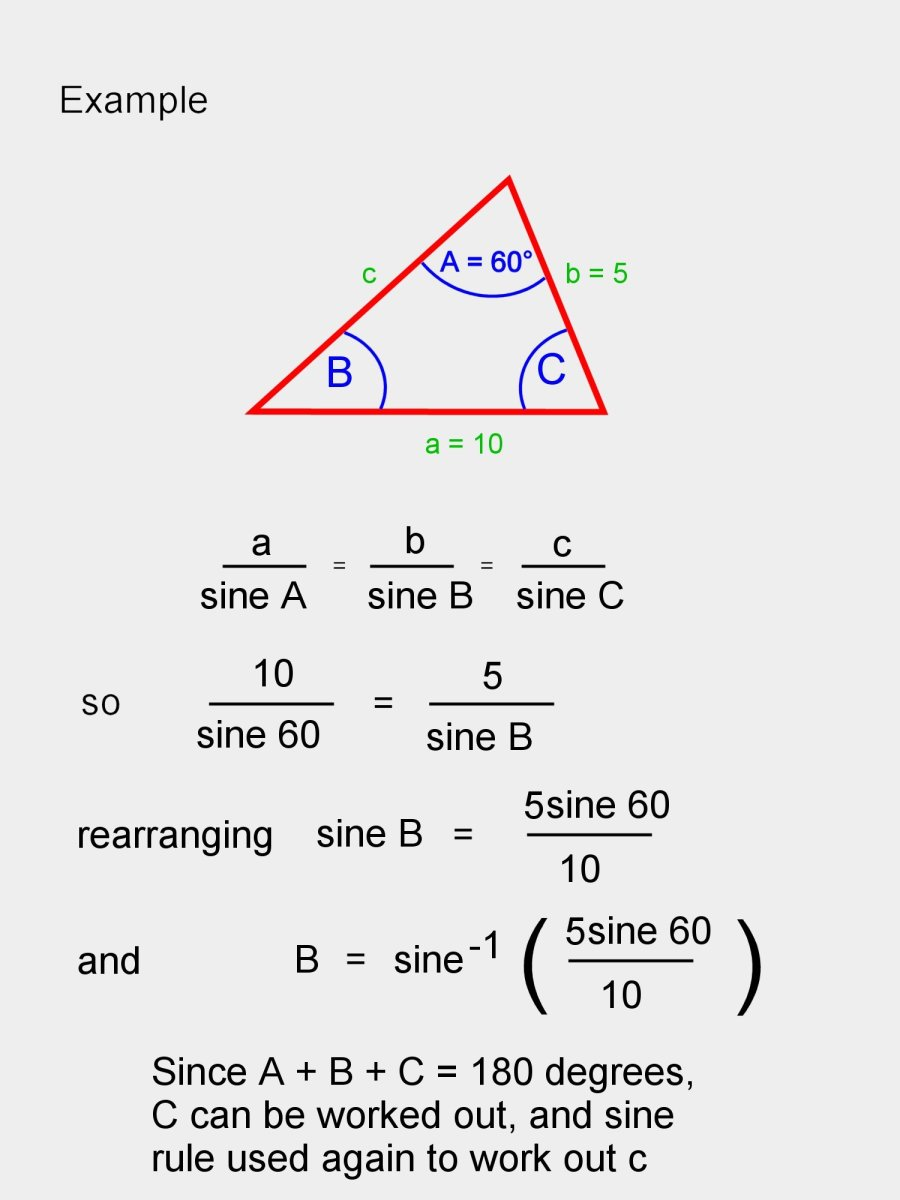Example showing how to use the sine rule to calculate the unknown side c.© Eugene Brennan

## The Cosine Rule

For a triangle with sides a, b, and c, if a and b are known and C is the included angle (the angle between the sides), C can be worked out with the cosine rule. The formula is as follows:

c = a2 + b2 - 2ab cos C

### When should the cosine rule be used?

1. You know the lengths of the two sides of a triangle and the included angle. You can then work out the length of the remaining side using the cosine rule.
2. You know the lengths of all the sides but none of the angles. Rearranging the cosine rule equation gives the length of one of the sides.

c = a2 + b2 - 2ab cos C

Rearranging the equation:

C = arccos ((a2 + b2 - c2) / 2ab)

The other angles can be worked out similarly.

## How to Find the Angles of a Triangle Knowing the Ratio of the Side Lengths

If you know the ratio of the side lengths, you can use the cosine rule to work out two angles then the remaining angle can be found knowing all angles add to 180 degrees.

Example:

A triangle has sides in the ratio 5:7:8. Find the angles.

So call the sides a, b and c and the angles A, B and C and assume the sides are a = 5 units, b = 7 units and c = 8 units. It doesn't matter what the actual lengths of the sides are because all similar triangles have the same angles. So if we work out the values of the angles for a triangle which has a side a = 5 units, it gives us the result for all these similar triangles.

Use the cosine rule. So c2 = a2 + b2 - 2ab cos C

Substitute for a,b and c giving:

8² = 5² + 7² - 2(5)(7) cos C

Working this out gives:

64 = 25 + 49 - 70 cos C

Simplifying and rearranging:

cos C = 1/7 and C = arccos(1/7).

You can use the cosine rule again or sine rule to find a second angle and the third angle can be found knowing all the angles add to 180 degrees.

## How to Get the Area of a Triangle

There are three methods that can be used to discover the area of a triangle.

### Method 1. Using the perpendicular height

The area of a triangle can be determined by multiplying half the length of its base by the perpendicular height. Perpendicular means at right angles. But which side is the base? Well, you can use any of the three sides. Using a pencil, you can work out the area by drawing a perpendicular line from one side to the opposite corner using a set square, T-square, or protractor (or a carpenter's square if you're constructing something). Then, measure the length of the line and use the following formula to get the area:

Area = 1/2ah

"a" represents the length of the base of the triangle and "h" represents the height of the perpendicular line.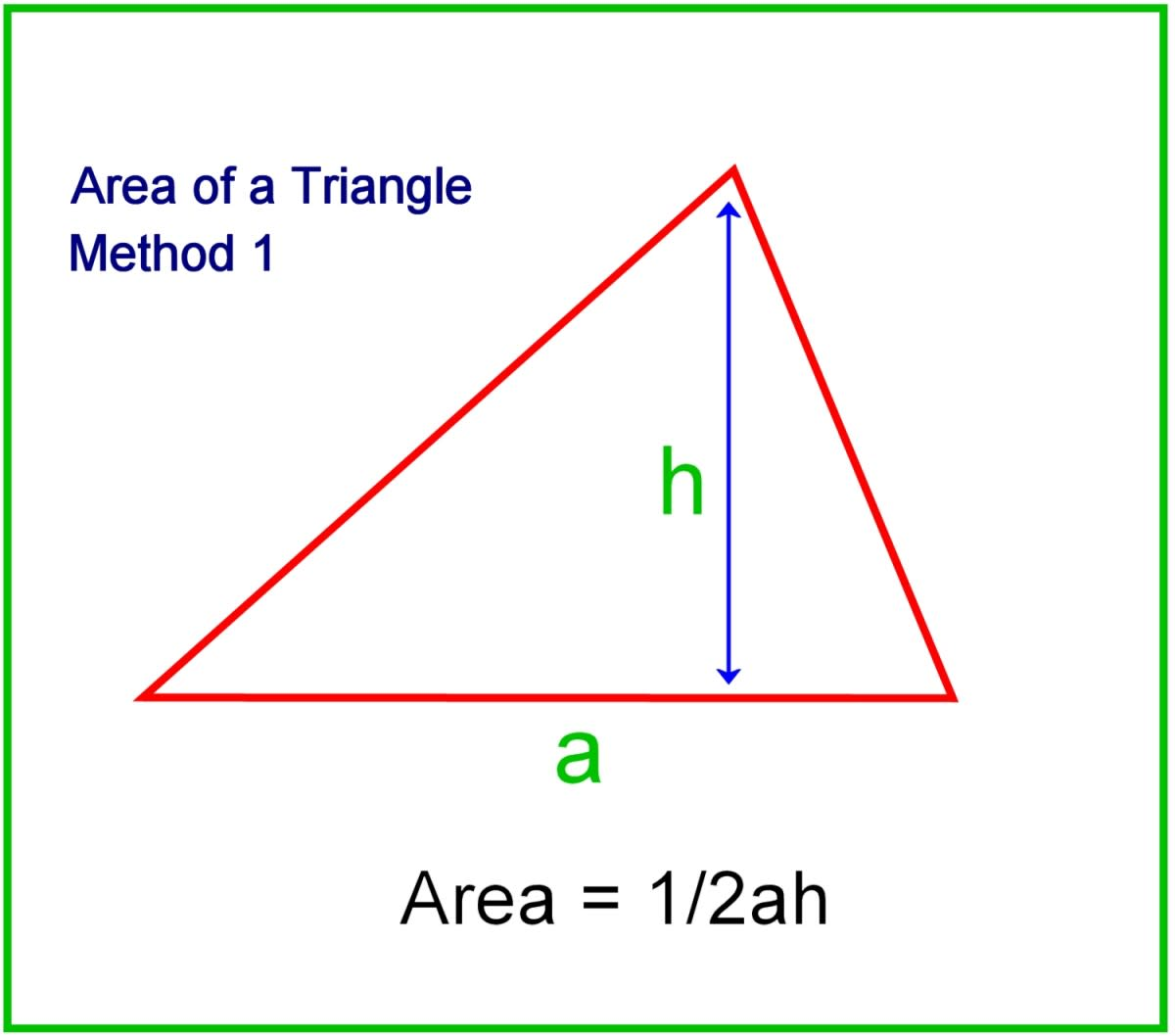Working out the area of a triangle from the base lengtth and perpendicular height.© Eugene Brennan

### Method 2. Using side lengths and angles

The simple method above requires you to actually measure the height of a triangle. If you know the length of two of the sides and the included angle, you can work out the area analytically using sine and cosine (see diagram below).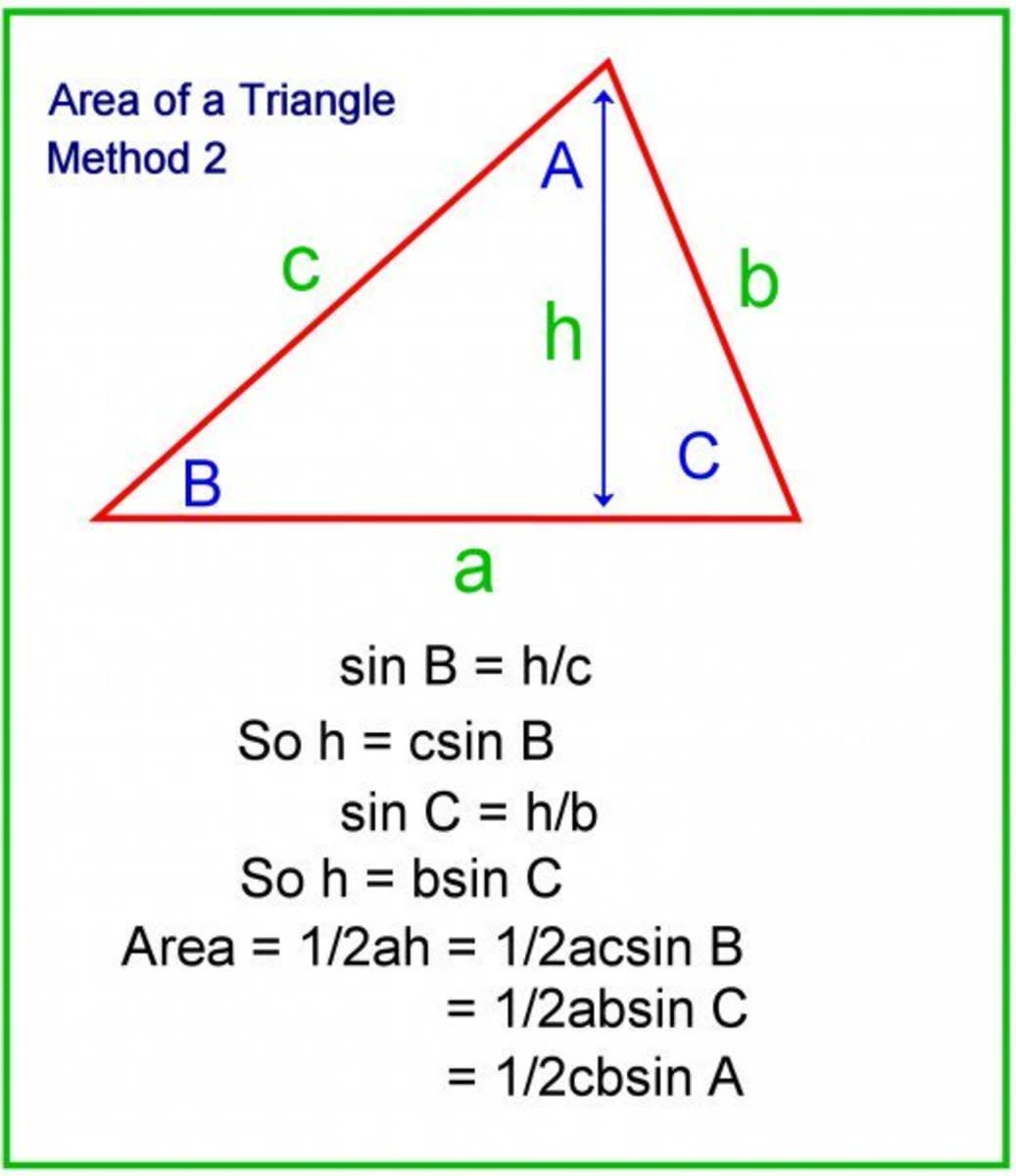Working out the area of a triangle from the lengths of two sides and the sine of the included angle.© Eugene Brennan

### Method 3. Use Heron's formula

All you need to know are the lengths of the three sides.

Area = √(s(s - a)(s - b)(s - c))

Where s is the semiperimeter of the triangle

s = (a + b + c)/2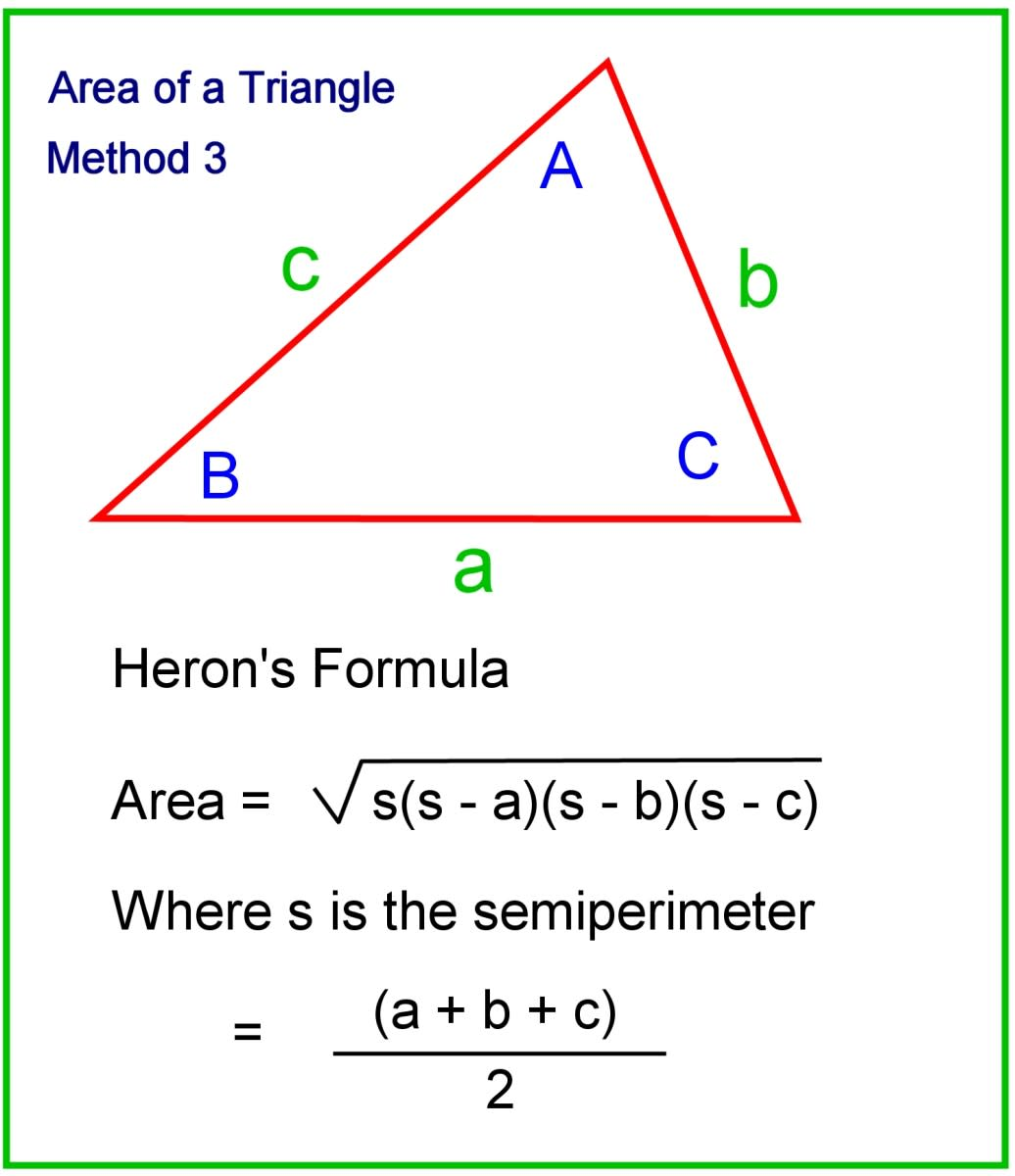Using Heron's formua to work out the area of a triangle.© Eugene Brennan

## Summary

If you've made it this far, you've learned numerous helpful methods to discover different aspects of a triangle. With all this information, you may be confused as to when you should use which method. The table below should help you identify which rule to use depending on the parameters you have been given.

## Find the Angles and Sides of a Triangle: Which Rule Do I Use?

A summary of how to work out angles and sides of a triangle.

Known ParametersTriangle TypeRule to Use

Triangle is right and I know length of two sides.

SSS after Pythagoras's Theorem used

Use Pythagoras's Theorem to work out remaining side and sine rule to work out angles.

Triangle is right and I know the length of one side and one angle

AAS after third angle worked out

Use the trigonometric identities sine and cosine to work out the other sides and sum of angles (180 degrees) to work out remaining angle.

I know the length of two sides and the angle between them.

SAS

Use the cosine rule to work out remaining side and sine rule to work out remaining angles.

I know the length of two sides and the angle opposite one of them.

SSA

Use the sine rule to work out remaining angles and side.

I know the length of one side and all three angles.

AAS

Use the sine rule to work out the remaining sides.

I know the lengths of all three sides

SSS

Use the cosine rule in reverse to work out each angle. C = Arccos ((a² + b² - c²) / 2ab)

I know the length of a side and the angle at each end

AAS

Sum of three angles is 180 degrees so remainging angle can be calculated. Use the sine rule to work out the two unknown sides

I know the length of a side and one angle

You need to know more information, either one other side or one other angle. Thes exception is if the known angle is in a rightangled triangle and not the right angle.

## FAQs About Triangles

Below are some frequently asked questions about triangles.

### What do the angles of a triangle add up to?

The interior angles of all triangles add up to 180 degrees.

### What Is the hypotenuse of a triangle?

The hypotenuse of a triangle is its longest side.

### What do the sides of a triangle add up to?

The sum of the sides of a triangle depends on the individual lengths of each side. Unlike the interior angles of a triangle, which always add up to 180 degrees

### How do you calculate the area of a triangle?

To calculate the area of a triangle, simply use the formula:

Area = 1/2ah

"a" represents the length of the base of the triangle. "h" represents its height, which is discovered by drawing a perpendicular line from the base to the peak of the triangle.

### How do you find the third side of a triangle that Is not right?

If you know two sides and the angle between them, use the cosine rule and plug in the values for the sides b, c, and the angle A.

Next, solve for side a.

Then use the angle value and the sine rule to solve for angle B.

Finally, use your knowledge that the angles of all triangles add up to 180 degrees to find angle C.

### How do you find the missing side of a right angled triangle?

Use the Pythagorean theorem to find the missing side of a triangle. The formula is as follows:

c2 = a2 + b2

c = √(a2 + b2)

### What is the name of a triangle with two equal sides?

A triangle with two equal sides and one side that is longer or shorter than the others is called an isosceles triangle.

### What is the cosine formula?

This formula gives the square on a side opposite an angle, knowing the angle between the other two known sides. For a triangle, with sides a, b and c and angles A, B and C the three formulas are:

a2 = b2 + c2 - 2bc cos A

or

b2 = a2 + c2 - 2ac cos B

or

c2 = a2 + b2 - 2ab cos C

### How to figure out the sides of a triangle if I know all the angles?

You need to know at least one side, otherwise, you can't work out the lengths of the triangle. There's no unique triangle that has all angles the same. Triangles with the same angles are similar but the ratio of sides for any two triangles is the same.

### How to work out the sides of a triangle if I know all the sides?

Use the cosine rule in reverse.
The cosine rule states:

c2 = a2 + b2 - 2ab cos C

Then, by rearranging the cosine rule equation, you can work out the angle

C = arccos ((a2 + b2 - c2) / 2ab)

and

B
= arccos ((a2+ c2 - b2) / 2ac)

The third angle A is (180 - C - B)

### How to find the perimeter of a triangle

Finding the perimeter of a triangle is a straightforward operation. The perimeter is equivalent to the added lengths of all three sides.

perimeter = a + b + c

### How to find the height of a triangle

Finding the height of a triangle is easy if you have the triangle's area. If you're given the area of the triangle:

height = 2 x area / base

If you don't have the area, but only have the side lengths of the triangle, use the following:

height = 0.5 x √ ((a + b + c)(-a + b + c)(a - b + c)(a + b - c)) / b

If you only have two sides and the angle between them, try this formula:

area = 0.5 (a)(b)(sin(γ)), then

height = area(sin(γ))

## Triangles in the Real World

A triangle is the most basic polygon and can't be pushed out of shape easily, unlike a square. If you look closely, triangles are used in the designs of many machines and structures because the shape is so strong.

The strength of the triangle lies in the fact that when any of the corners are carrying weight, the side opposite acts as a tie, undergoing tension and preventing the framework from deforming. For example, on a roof truss, the horizontal ties (which can be joists in a ceiling) provide strength and prevent the roof from spreading out at the eaves.

The sides of a triangle can also act as struts, but in this case, they undergo compression. An example is a shelf bracket or the struts on the underside of an airplane wing or the tail wing itself.